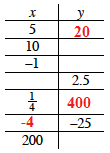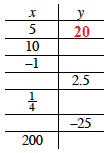### Home > MC1 > Chapter 10 > Lesson 10.2.2 > Problem10-85

10-85.

Copy and complete the table below with values of x and y that work for the rule xy = 100.

x

y

5

10

−1

2.5

$\frac { 1 } { 4 }$

−25

200

5(y) = 100

$\frac{100}{5}=20$

y = 20